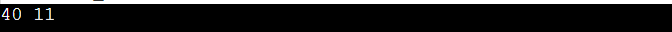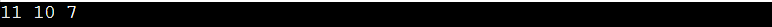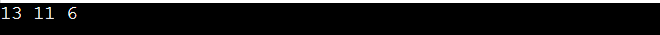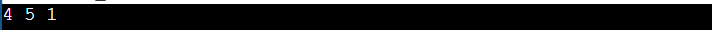New update is available. Click here to update.
Last Updated: Jun 30, 2023
Medium

# Find duplicates in a given array when elements are not limited to a range0 upvotes

## Introduction

In this article, we will  find the duplicate number in a limited range array of size n with elements between 1 and n-1.

Now let us understand the concept of finding duplicates with the help of an example.

## Approach: Using Hashing

As we know, a data structure that associates values with keys is known as a hash table. This calculates key indexes using a hash function. We can store the value at the proper location based on the Hash Table index.

Now that you've devised a concept, let's look at its algorithm and implementation:

### Approach: Use unordered_map for hashing

Count the number of times each element appears, and the elements with a frequency greater than one are printed. Because the range of numbers is unknown, unordered_map is used.

### Implementation in Java

``````/*Java program to find duplicates in the array given*/
import java.util.HashMap;
import java.util.Map;
import java.util.Map.Entry;

public class Main
{
// Driver program
public static void main(String[] arg)
{
int array[] = {11, 10, 40, 11, 7, 9, 5, 40, 11};
int n = array.length;
printingDuplicates(array, n);
}
//finding and printing duplicates
private static void printingDuplicates(int[] array, int n)
{
Map<Integer,Integer> map = new HashMap<>();
int count = 0;
boolean dupl = false;
for(int i = 0; i < n; i++){
if(map.containsKey(array[i])){
count = map.get(array[i]);
map.put(array[i], count + 1);
}
else{
map.put(array[i], 1);
}
}

for(Entry<Integer,Integer> entry : map.entrySet())
{
/* if the frequency is greater than 1
printing the element */
if(entry.getValue() > 1){
System.out.print(entry.getKey()+ " ");
dupl = true;
}
}
// if no duplicates are present
if(!dupl){
System.out.println("-1");
}
}
}``````

### Output### Implementation in C++

``````#include <bits/stdc++.h>
using namespace std;

// function duplicates to find and print
void Duplicates(int arry[], int n)
{
// using unordered_map to store frequencies
unordered_map<int, int> freqc;
for (int i=0; i<n; i++)
freqc[arry[i]]++;

bool dupl = false;
unordered_map<int, int>:: iterator itr;
for (itr=freqc.begin(); itr!=freqc.end(); itr++)
{
// if the frequency is more than 1 print element
if (itr->second > 1)
{
cout << itr->first << " ";
dupl = true;
}
}

//If duplicates are not present
if (dupl == false)
cout << "-1";
}

// to test the driver program
int main()
{
int arry[] = {7, 18, 10, 10, 7, 6, 11, 12, 11};
int n = sizeof(arry) / sizeof(arry);
Duplicates(arry, n);
return 0;
}``````

### Output### Implementation in Python3

To store a number as a key and its frequency as a value in Python, use a dictionary. Because the range of integers is unknown, a dictionary can be used.

``````# Python3 program for finding duplicates
# by using a dictionary approach.
def printingDuplicates(arry):
dict = {}

for elem in arry:
try:
dict[elem] += 1
except:
dict[elem] = 1

for itm in dict:

# if the frequency is greater than 1
# then printing  the element
if(dict[itm] > 1):
print(itm, end=" ")

print("\n")

# Driver Code
if __name__ == "__main__":
list = [13, 13, 11, 6,
2, 6, 5, 12, 11]
printingDuplicates(list)``````

### Output### Implementation in C#

``````/* C# program for finding the duplicates in given array*/
using System;
using System.Collections.Generic;

class findingDuplicates
{
// function for finding and printing duplicates
static void printingDuplicates(int[] arry, int n)
{
Dictionary<int,
int> map = new Dictionary<int,
int>();
int count = 0;
bool dupl = false;
for (int i = 0; i < n; i++)
{
if (map.ContainsKey(arry[i]))
{
count = map[arry[i]];
map[arry[i]]++;
}
else
}

foreach (KeyValuePair<int,
int> entry in map)
{
// if the frequency is greater than 1
// printing the element
if (entry.Value > 1)
Console.Write(entry.Key + " ");
dupl = true;
}

// if no duplicates found
if (!dupl)
Console.WriteLine("-1");
}

// Driver Code
public static void Main(String[] arg)
{
int[] arry = { 3, 4, 4, 7,
5, 4, 5, 1, 1 };
int n = arry.Length;
printingDuplicates(arry, n);
}
}``````

### OutputThe above solution has time complexity O(N) and auxiliary space is  O(N)

### In an array, how do you find repeated elements?

To find the repeated elements you first need to make a new array and initialise it with data.The we need two loops to find duplicate elements, where the outer loop iterates across the array from 0 to the array's length. If a match is detected, it indicates the duplicate element has been found, so display it.

### How do you use a for loop to locate duplicates in an array?

In brute force approach for loop will be used to sort and iterate duplicates will be adjacent to each other and easy to detect after the array has been sorted.In a HashSet approach, we can populate this while iterating & abort if a match is found.As the for loop will run for n time it will have O(n) time and O(n) space complexity on average.

### Is it true that the spread operator removes duplicates?

Remove duplicate elements and sort an array of numbers and objects using the spread operator without destroying the original array.

### How do you search an array for unique elements?

There are several ways to find unique elements from an array such as by storing all of the elements in the key of the hashmap, using the nested loop technique or by sorting is an effective method and by the use of hashing.

### Duplicates in an array are removed in what way?

There are two ways to remove duplicate elements from an array. One of it is using a temporary array or using a distinct index. The array must be in sorted order in order to delete the duplicate element. If the array is not sorted, use the Arrays. sort(arr) method to sort it.

## Conclusion

In this blog we have discussed approaches in C++, Java, Python3 and C# using unordered hashmap ,to find duplicates in a given array when elements are not limited to a range. You can also look at more exciting blogs and articles curated by the coding ninja's team by visiting the Library.

We hope that this blog has helped you enhance your knowledge regarding finding duplicates in arrays in C++ and if you would like to learn more, check out our articles on Arrays in C++.

Check out the following problems -Refer to our guided paths on Coding Ninjas Studio to learn more about DSA, Competitive Programming, JavaScript, System Design, etc. Enrol in our courses and refer to the mock test and problems available, Take a look at the interview experiences and interview bundle for placement preparations.

Do upvote our blog to help other ninjas grow.

Happy Learning!